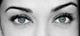# Math Is Fun Forum

Discussion about math, puzzles, games and fun.   Useful symbols: ÷ × ½ √ ∞ ≠ ≤ ≥ ≈ ⇒ ± ∈ Δ θ ∴ ∑ ∫  π  -¹ ² ³ °

You are not logged in.

## #1 2006-12-26 09:33:18

unique
MemberRegistered: 2006-10-04
Posts: 419

### LINe

Write the equation of the line that is equidistant from the points (4, 2) and (-4, 3).

distance (x, y) to (-4, 3) = distance (x, y) to (4, 2)

----------------------------------------------------------

sqrt( [(x) - (-4)]^2 + [(3) - (y)]^2 ) = sqrt( [(4) - (x)]^2 + [(2) - (y)]^2 )

(x + 4)^2 + (3 - y)^2 = (4 - x)^2 + (2 - y)^2

x^2 + 16x + 16 + 9 - 6y + y^2 = 16 - 8x + x^2 + 4 - 4y + y^2

16x + 16 + 9 - 6y = 16 - 8x + 4 - 4y

24x + 10y - 5 = 0

y = - 12/5x + 5/10

Desi
Raat Key Rani !

Offline

## #2 2006-12-26 09:54:14

luca-deltodesco
MemberRegistered: 2006-05-05
Posts: 1,470

### Re: LINe

the way to do this would be to see that this line would be a perpendicular bisector of the line joining the points that passes through the middle.

imagining this line between the two points, its gradient would be

so the gradient of the line we want would be

the midpoint of this line would be

so our line is

you can ofcourse just solve algebraicly,

youre errors came in in changing the right side to 0 and in the expansion of the squared terms

Last edited by luca-deltodesco (2006-12-26 10:01:05)

The Beginning Of All Things To End.
The End Of All Things To Come.

Offline